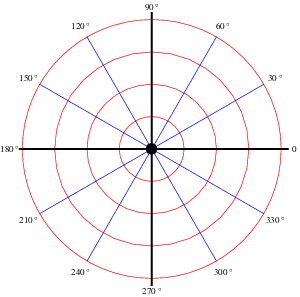# Coordinates of the Turtle World¶

In the turtle world, we use a coordinate system as the following:

1. The origin (0,0) is at the center of the graphics window.
2. The x axis grows from left to right.
3. The y axis grows from bottom to top.## Set the turtle’s position¶

setxy(x,y) set the turtle’s position to point(x,y) without a trace.

gotoxy(x,y) move the turtle to point(x,y). So if the pen is down, there will be a trace.

The turtle’s heading direction will not change.

The following program demonstrated the result of gotoxy() and setxy().

```from easygraphics.turtle import *

def main():
create_world(300,300)
gotoxy(50,-100)
for i in range(360):
fd(1)
lt(1)
pause()
cs()

setxy(50,-100)
for i in range(360):
fd(1)
lt(1)

pause()
close_world()

easy_run(main)
```

facing(x,y) set the turtle heading to facing point(x,y).

```from easygraphics.turtle import *

def main():
create_world(300,300)

# set the turtle's heading to top-left corner of the graphics window
facing(-150,150)
fd(100)

pause()
close_world()

easy_run(main)
```

set_heading(angle) set the turtle’s new orientation to angle.

Values of the angles in the turtle world are as the following:```from easygraphics.turtle import *

def main():
create_world(300,300)

# set the turtle's heading to top-left corner of the graphics window
fd(100)

pause()
close_world()

easy_run(main)
```

## Get turtle’s current state¶

get_x() return the x coordinate value of the turtle’s position.

get_y() return the y coordinate value of the turtle’s position.

```from easygraphics.turtle import *

def main():
create_world(300,300)

# set the turtle heading to top-left corner of the graphics window
facing(-150,150)
fd(100)